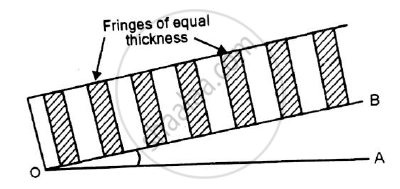# Explain how interference in wedge shaped film is used to test optical flatness of given glass plate. - Applied Physics 2

Short Note

Explain how interference in wedge shaped film is used to test optical flatness of given glass plate.

#### Solution

1.The phenomenon of interference is used in testing the plainness of the surface of glass plate.2.If two surfaces OA and OB are perfectly plane,the air film between them would gradually vary in thickness from O to A.The fringes are of equal thickness as each fringe is the locus of points at which the thickness of the film has a constant value.
3.To test the optical flatness of a surface,the specimen surface to be tested(OB) is placed overan optically plane surface (OA).
4.The fringes are observed in the field of view.
5.If the fringes are of equal thickness then surface is plane.
6.If the fringes are not of equal thickness then surface is not plane.

7.In this way interference in wedge shaped film is used to test optical flatness of given glass plate.

Concept: Wedge Shaped Film(Angle of Wedge and Thickness Measurement)
Is there an error in this question or solution?